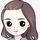cancel
Showing results for
Did you mean:

Fabric is Generally Available. Browse Fabric Presentations. Work towards your Fabric certification with the Cloud Skills Challenge.Helper I

## Need Rank based on summarized value instead of Don't Summarize.

Hi Community,

I'm creating a table visual, having columns Unique_id, date, Sales, Quantity, UOM and a measure Unit Price.

Unit Price=  Divide(sum(Sales),sum(quantity))

Now I want to create a Rank Value based on Unit Price Measure, using below measure:

Rank= rankx(allselected('Table',[Unit Price], ,ASC)

When I pull this measure not getting correct value of rank.

When I do, don't summarize for Sales and Quantity in Values Pane , the value comes correct, but I need rank based summarization of Sales and Quantity. How to correct this Rank Value...

1 ACCEPTED SOLUTIONCommunity Support

Hi @mg01 ,

I updated your sample pbix file(see the attachment), please check whether that is what you want.

1. Update the formula of measure [Unit Price] as below

``````Unit Price =
VAR _seluid =
SELECTEDVALUE ( 'Table'[Unique_id] )
VAR _seluom =
SELECTEDVALUE ( 'Table'[UOM] )
VAR _seldate =
SELECTEDVALUE ( 'Table'[date] )
VAR _sumsales =
CALCULATE (
SUM ( 'Table'[Sales] ),
FILTER (
ALLSELECTED ( 'Table' ),
'Table'[Unique_id] = _seluid
&& 'Table'[UOM] = _seluom
&& 'Table'[date] = _seldate
)
)
VAR _sumqty =
CALCULATE (
SUM ( 'Table'[Quantity] ),
FILTER (
ALLSELECTED ( 'Table' ),
'Table'[Unique_id] = _seluid
&& 'Table'[UOM] = _seluom
&& 'Table'[date] = _seldate
)
)
RETURN
DIVIDE ( _sumsales, _sumqty, 0 ) //DIVIDE(sum('Table'[Sales]),sum('Table'[Quantity]))``````

2. Update the formula of measure [] as below

 Rank = RANKX ( ALLSELECTED ( 'Table' ), [Unit Price],, ASC, DENSE )Best Regards

Community Support Team _ Rena
If this post helps, then please consider Accept it as the solution to help the other members find it more quickly.
4 REPLIES 4Super User

Hi,

``Rank 2 = if(isblank([Unit Price]),blank(),RANKX(filter(generate(all('Calendar'[Date]),generate(ALL('Table'[Unique_id]),all('Table'[UOM]))),[Total quantity]<>blank()),[Unit Price],,ASC))``

Hope this helps.Regards,
Ashish Mathur
http://www.ashishmathur.comSuper User

@mg01 , Rank can change based on context

Lowset level of table

Rank= rankx(allselected('Table') ,[Unit Price], ,ASC)

for product, any other column will give rank of product inside that column

Rank= rankx(allselected('Table'[Product]) ,[Unit Price], ,ASC)

Two columns

Rank= rankx(allselected('Table'[Product], 'Table'[Cagegory]) ,[Unit Price], ,ASC)Helper I

Only single column can be passed under allselected, I tried all option you shared. Can you please tried with shared file and any suggestion at top of it.Community Support

Hi @mg01 ,

I updated your sample pbix file(see the attachment), please check whether that is what you want.

1. Update the formula of measure [Unit Price] as below

``````Unit Price =
VAR _seluid =
SELECTEDVALUE ( 'Table'[Unique_id] )
VAR _seluom =
SELECTEDVALUE ( 'Table'[UOM] )
VAR _seldate =
SELECTEDVALUE ( 'Table'[date] )
VAR _sumsales =
CALCULATE (
SUM ( 'Table'[Sales] ),
FILTER (
ALLSELECTED ( 'Table' ),
'Table'[Unique_id] = _seluid
&& 'Table'[UOM] = _seluom
&& 'Table'[date] = _seldate
)
)
VAR _sumqty =
CALCULATE (
SUM ( 'Table'[Quantity] ),
FILTER (
ALLSELECTED ( 'Table' ),
'Table'[Unique_id] = _seluid
&& 'Table'[UOM] = _seluom
&& 'Table'[date] = _seldate
)
)
RETURN
DIVIDE ( _sumsales, _sumqty, 0 ) //DIVIDE(sum('Table'[Sales]),sum('Table'[Quantity]))``````

2. Update the formula of measure [] as below

 Rank = RANKX ( ALLSELECTED ( 'Table' ), [Unit Price],, ASC, DENSE )Best Regards

Community Support Team _ Rena
If this post helps, then please consider Accept it as the solution to help the other members find it more quickly.Announcements#### Power BI Monthly Update - November 2023

Check out the November 2023 Power BI update to learn about new features.#### Fabric Community News unified experience

Read the latest Fabric Community announcements, including updates on Power BI, Synapse, Data Factory and Data Activator.#### Exclusive opportunity for Women!

Join us for a free, hands-on Microsoft workshop led by women trainers for women where you will learn how to build a Dashboard in a Day!#### The largest Power BI and Fabric virtual conference

130+ sessions, 130+ speakers, Product managers, MVPs, and experts. All about Power BI and Fabric. Attend online or watch the recordings.Top Solution Authors
Top Kudoed Authors
Users online (3,975)